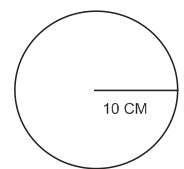# 0.27 g of a long chain fatty acid was dissolved in 100 cm3 of hexane. 10 mL of this solution was added dropwise to the surface of water in a round watch glass. Hexane evaporates and a monolayer is formed. The distance from edge to centre of the watch glass is 10 cm. What is the height of the monolayer?[Density of fatty acid=0.9 g cm-3 ,

Density is a measure of mass per unit  volume. The average density of an object is equals to its total mass divided by its total volume. An object made from a comparatively dense material (such as iron) will have less volume than an object of equal mass made from some less dense substance (such as water).

To find the density of any object, we need to know the Mass (grams) of the object, and its Volume (measured in mL or cm³). Divide the mass by the volume in order to get an object's Density.

D = m/v.

-

1 cm3 = 1 mL

100 cm3 = 100 mL

Then 10 mL of hexanme contains=Distance from edge to centre of watch glass=10 cm

height of monolayer=?

The volume of fatty acid over glass plate=

Volume = Area X height

So, height of monolayer=10-4 cm

=10-4 X 10-2

=10-6 m

### Preparation Products

##### Knockout JEE Main Sept 2020

An exhaustive E-learning program for the complete preparation of JEE Main..

₹ 12999/- ₹ 6999/-
##### Rank Booster JEE Main 2020

This course will help student to be better prepared and study in the right direction for JEE Main..

₹ 9999/- ₹ 4999/-
##### Test Series JEE Main Sept 2020

Take chapter-wise, subject-wise and Complete syllabus mock tests and get in depth analysis of your test..

₹ 4999/- ₹ 1999/-
##### Knockout JEE Main April 2021

An exhaustive E-learning program for the complete preparation of JEE Main..

₹ 22999/- ₹ 14999/-